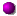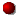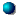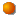# \$B%h%sWB@1(B

172P/Yeung###\$B2s5"5-O?(B\$BH/8+(B\$B=P8=(B\$B4QB,\$5\$l\$J\$+\$C\$?2s5"(B\$BH/8+A0\$N=P8=(B\$BH/8+A0\$N4QB,\$5\$l\$J\$+\$C\$?2s5"(B\$B>-Mh\$N2s5"(B172P 2017/03/13172P 2008/10/12172P/2002 BV 2002/03/11172P 1995/08/02##### 1995\$BG/\$N6aF|E@DL2aF|\$OB<2,7r<#;a\$N7W;;\$K\$h\$k\$b\$N!#(B 1995\$BG/\$N=P8=\$O(BM.P.E.C. 2002-J34\$B\$*\$h\$S(BNK 1224\$B\$K7G:\\$5\$l\$?\$b\$N!#(B